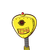# If the velocity of a body increases by threetimes the ate for previous change in kinetic energya) 1500% b) 800% c) 15%

If the velocity of a body increases by three
times the ate for previous change in kinetic energy
a) 1500% b) 800% c) 15% d)900% %​

### 1 thought on “If the velocity of a body increases by three<br />times the ate for previous change in kinetic energy<br />a) 1500% b) 800% c) 15%”

1.• Initial velocity = v
• Final velocity = v + 3v = 4v
• Mass = m

Kinetic Energy :

• It’s always non negative.
• It’s a scalar quantity.
• It depends on frame of reference.
• It doesn’t depend on direction of velocity.

Formula for calculating the Kinetic energy :

• K.E = ½mv²

According to the Question :

=> ∆K.E % = [(K.Ef – K.Eᵢ)/K.Eᵢ] × 100

=> ∆K.E % = [(½m(4v)² – ½m(v)²)/½m(v)²] × 100

=> ∆K.E % = [(16v² – v²)/v²] × 100

=> ∆K.E % = (15v²/v²) × 100

=> ∆K.E % = 15 × 100

=> ∆K.E % = 1500 %

• Hence,the option A) 1500% is the correct answer.